# Electronics and Communication Engineering - Electromagnetic Field Theory

11.
A 10 GHz signal propagates in a waveguide with a phase velocity of 4 x 108 m/s. If the signal produces standing wave in the guide, then the distance between successive minimal will be
10 mm
20 mm
30 mm
40 mm
Explanation:

f = 10 GHz, v = 4 x 108 m/sec,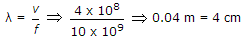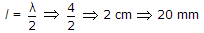.

12.
The incidence from dielectric medium 1 (ε1) into dielectric medium 2 (ε2), Brewster angle θB and the corresponding angle of transmission θt for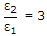will be respectively
30° and 30°
30° and 60°
60° and 30°
30° and 60°
Explanation:

Brewster angle θ = tan-1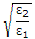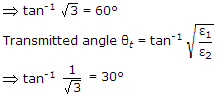13.
A medium of relative permittivity εr2 = 2 forms an interface with free space. A point source of EM energy is located in the medium at a depth of 1 meter from the interface. Due to the total internal reflection, the transmitted beam has a circular cross-section over the interface. The area of the beam cross section at the interface is given by
2p m2
p2 m2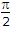m2
p m2
Explanation: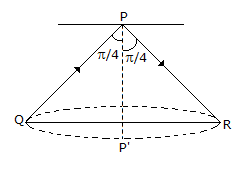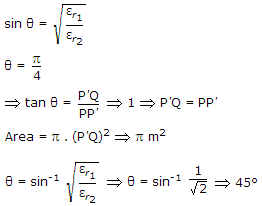14.
A transmission line of characteristic impedance z0 = 50 Ω. Phase Velocity = 2 x 108 m/sec, and length l = 1 m is terminated by a load z1 = (30 - j 40) ohms. The input impedance of the line for a frequency of 100 MHz will be
(30 + j x 40) Ω
(30 - j 40) Ω
(50 + j x 40) Ω
(50 - j 40) Ω
Explanation: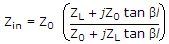.

15.
A circular waveguide carries TE11 mode whose radial electric-field is given by
Er = E 0J1 (r) sin φ V/m
where 'r' is the radial distance in cm, from the waveguide axis. The cut-off wavelength of the mode is
10 cm
3p cm
2p cm
8 cm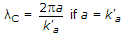.